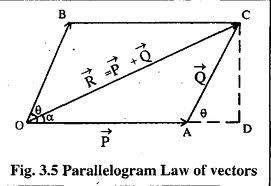# Angle between two vectors book problem

• B
• gracy

#### gracyShould not angle between vector P and Q be 180-θ rather than θ? According to my book it is θ.

If you put them tail to tail you can see that the angle is theta. Actually OB is the vector Q translated so that it has same origin as P.
Another way to look at it is that the projection of Q along P is in the positive direction so the angle should be less than 90 degrees.
All this assumes that the vector Q is from A to C. It does not have an arrow in the drawing.

gracy said:
View attachment 100320
Should not angle between vector P and Q be 180-θ rather than θ? According to my book it is θ.
The parallelogram is just a 'construction'. The vectors are OA and OB, which makes the angle between them θ. Another way of looking at it is to consider the difference between the respective angles of each vector and the X axis (or any other axis).

## 1. What is the angle between two vectors?

The angle between two vectors is the measure of the smallest angle needed to rotate one vector onto the other. It is typically measured in degrees or radians and can range from 0 to 180 degrees.

## 2. How do I find the angle between two vectors?

To find the angle between two vectors, you can use the dot product formula:
θ = cos-1(a · b / |a||b|)
where a and b are the two vectors and |a| and |b| are their magnitudes. Alternatively, you can use trigonometric functions such as sine and cosine to calculate the angle.

## 3. What is the difference between acute and obtuse angles?

An acute angle is less than 90 degrees, while an obtuse angle is greater than 90 degrees. In the context of finding the angle between two vectors, an acute angle indicates that the two vectors are pointing in similar directions, while an obtuse angle indicates that they are pointing in opposite directions.

## 4. Can the angle between two vectors be negative?

No, the angle between two vectors cannot be negative. It is always a positive value between 0 and 180 degrees. If you get a negative value, it means you have made an error in your calculations.

## 5. How can I use the angle between two vectors to solve problems?

The angle between two vectors can be used to solve a variety of problems in physics, engineering, and mathematics. For example, it can be used to find the work done by a force, the magnitude of a vector projection, or the direction of a resultant vector. It is a useful tool in analyzing and solving vector-based problems.